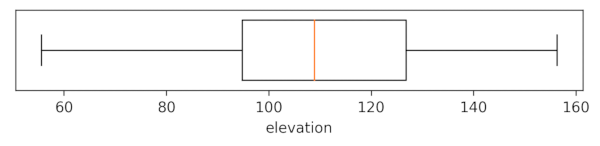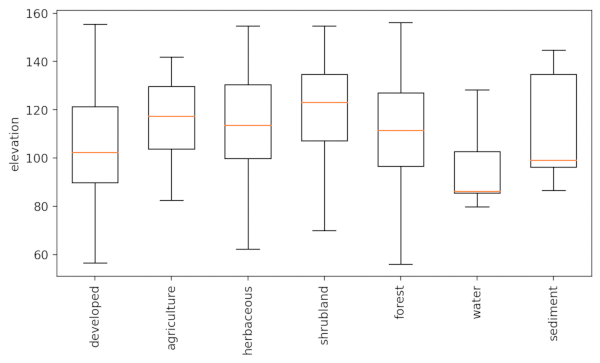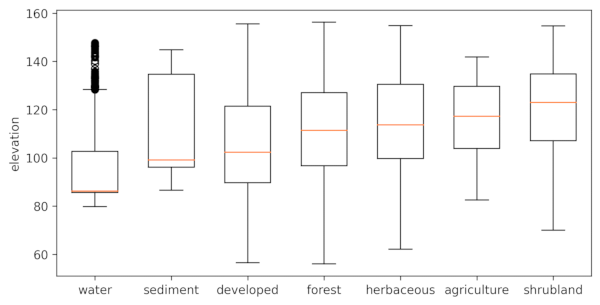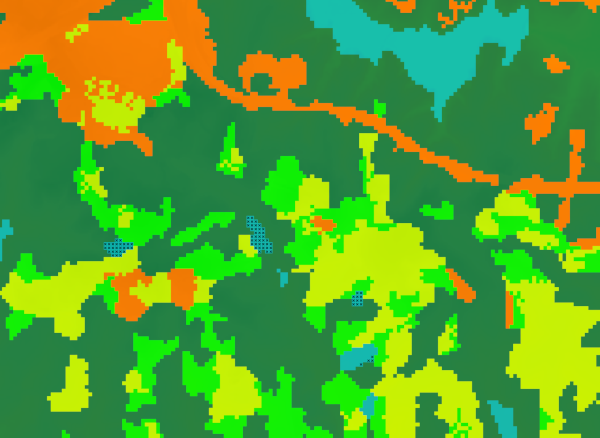## NAME

r.boxplot - Draws the boxplot of raster values. Optionally, this is done per category of a zonal raster layer

## KEYWORDS

display, raster, plot, boxplot

## SYNOPSIS

r.boxplot
r.boxplot --help
r.boxplot [-honrc] input=name [zones=name] [output=name] [order=string] [range=float] [map_outliers=string] [fontsize=integer] [plot_dimensions=string] [dpi=integer] [--overwrite] [--help] [--verbose] [--quiet] [--ui]

### Flags:

-h
horizontal boxplot(s)
Draw the boxplot horizontal
-o
include outliers
Draw boxplot(s) with outliers
-n
notch
Draw boxplot(s) with notch
-r
rotate labels
rotate labels
-c
Color boxploxs
Color boxploxs using the colors of the categories of the zonal raster
--overwrite
Allow output files to overwrite existing files
--help
Print usage summary
--verbose
Verbose module output
--quiet
Quiet module output
--ui
Force launching GUI dialog

### Parameters:

input=name [required]
input raster
input raster
zones=name
zonal raster
categorical map with zones
output=name
Name of output image file
Name for output file
order=string
Sort boxplots
Sort boxplots based on their median values
Options: descending, ascending
range=float
Range (value > 0)
this determines how far the plot whiskers extend out from the box. If range is positive, the whiskers extend to the most extreme data point which is no more than range times the interquartile range from the box. A value of zero causes the whiskers to extend to the data extremes.
Default: 1.5
map_outliers=string
Name of outlier map
Create a vector point layer of outliers
fontsize=integer
Font size
Default font size
plot_dimensions=string
Plot dimensions (width,height)
Dimensions (width,height) of the figure in inches
dpi=integer
DPI
resolution of plot

## DESCRIPTION

r.boxplot draws boxplots of the raster values of a input raster layer. The user has the option to define a zonal (categorical) layer. In that case, a boxplot will be drawn for each zone, using the values of the input raster that fall within that zone.

By default, the resulting plot is displayed on screen. However, the user can also save the plot to file using the output option. The format is determined by the extension given by the user. So, if output = outputfile.png, the plot will be saved as a png file.

The whiskers extend to the most extreme data point, which is no more than range ✕ the interquartile range (iqr) from the box. By default, a range of 1.5 is used, but the user can change this. Note that range values need to be larger than 0.

By default, outliers are not included in the plot. Set the -o flag to include them in the plot. To also create a point vector map with the locations of the outliers, the user needs to provide the name of the output map using map_outliers.

There are a few layout options, including the option to rotate the plot and the x-axis labels, print the boxplot(s) with notches, sort the boxplot from low to high (ascending) or from high to low (descending) median, and color the boxplots according to the corresponding categories of the zonal raster.

## NOTE

To include outliers, the function converts the raster cell with outlier values to a point vector layer. This may take some time if there are a lot of outliers. So, if you are working with very large raster layers, be cautious to not set the range value very low as that may result in a very large number of outliers.

## EXAMPLE

### Example 1

Draw a boxplot of the values of the elevation layer from the NC sample dataset. Set the -h flag to print the boxplot horizontally. Set the plot dimensions to 7 inch wide, 1 inch heigh.
```g.region raster=elevation
r.boxplot -h input=elevation plot_dimensions="7,1" output="r_boxplot_01.png"
```### Example 2

Draw boxplots of the values of the elevation layer per category from the landclass96 layer from the same NC sample dataset. Use the -r flag to rotate the x-asis labels.
```r.boxplot -r input=elevation zone=landclass96 output="r_boxplot_02.png"
```### Example 3

Draw boxplots of the values of the elevation layer per category from the landclass96 layer from the same NC sample dataset. Set the -o flag to include outliers. Use order=ascending to order the boxplots from low to high median. Provide a name for the outlier map to save the outlier locations as a point vector map.
```r.boxplot -o order=ascending input=elevation zones=landclass96 output="r_boxplot_03.png" map_outliers="outliers"
```Below, part of the landclass96 raster map is shown, with on top the vector point layer with location of outliers. Curiously, for some lakes, only part of the raster cells are outliers.### Example 4

Draw boxplots of the values of the elevation layer per category from the landclass96 layer from the same NC sample dataset. Set the -c flag to color the boxplots, use order=ascending to order the boxplots from low to high median, and set the fontsize to 11.
```r.boxplot -c order=ascending fontsize=11 input=elevation zones=landclass96 output="r_boxplot_04.png"
```## Acknowledgements

This work was carried in the framework of the Save the tiger, save the grassland, save the water project by the Innovative Bio-Monitoring research group.

r.scatterplot, r.stats.zonal, d.vect.colbp

## AUTHOR

Paulo van Breugel
Applied Geo-information Sciences
HAS University of Applied Sciences

## SOURCE CODE

Available at: r.boxplot source code (history)

Latest change: Sun May 8 19:37:05 2022 in commit: 00caf546639d5c8264ba3ad6f7637e404f14cc83

© 2003-2022 GRASS Development Team, GRASS GIS 8.0.3dev Reference Manual